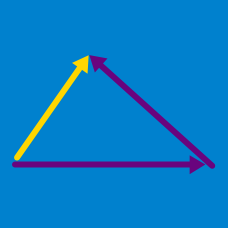Geometry

# Vector Decomposition

Find the magnitude of the vector $\vec{v}=(-3,4)?$

Point P lies on side BC and divides it internally in the ratio 1 : 4 in the equilateral triangle $ABC$ below. Let $\vec{AB}=\vec{a},$ $\vec{AC}=\vec{b},$ $\vec{AP}=\vec{c}.$ Then $\vec{c}$ can be expressed as $x\vec{a}+y\vec{b}.$ Which of the following is equal to $x\times y$?$G$ is the centroid of triangle $OAB$ below. Let $\vec{OA}=\vec{a}, \vec{OB}=\vec{b},$ and $\vec{GA}=\vec{c}$. Then $\vec{c}$ can be expressed as $x\vec{a}-y\vec{b}.$ Which of the following is equal to $x - y$?A commercial airliner can fly at a cruising velocity of 250m/s without the influence of the wind. If a 30m/s gust continuously blows 70$^\circ$ south of east, then how many degrees north of east should the pilot angle the plane thereby counteracting the speed of the wind so that the plane flies directly east?

NOTE: "South of east" and "north of east" are terms used in bearing; imagine a pointer straight east, and the rotates some angle clockwise (that's south of east) or counterclockwise (that's north of east).

Let the position vectors of three points $A, B,$ and $C$ be $\vec{a}, \vec{b},$ and $\vec{c},$ respectively. Then $2\vec{AB}+3\vec{BC}-\vec{CA}$ can be expressed as $x\vec{a}+y\vec{b}+z\vec{c}.$ Calculate $x^2+y^2+z^2$.

×